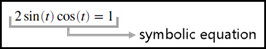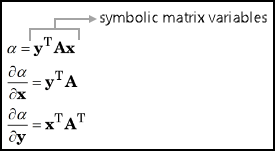Symbolic Objects to Represent Mathematical Objects

To solve mathematical problems with Symbolic Math Toolbox™, define symbolic objects to represent various mathematical objects. This example discusses the usage of the following symbolic objects:

• symbolic numbers

• symbolic scalar variables, functions, and expressions

• symbolic equations

• symbolic vectors and matrices

• symbolic matrix variables (since R2021a)

Symbolic Number

Defining a number as a symbolic number instructs MATLAB® to treat the number as an exact form instead of using a numeric approximation. For example, use a symbolic number to represent the argument of an inverse trigonometric function $\theta ={\mathrm{sin}}^{-1}\left(1/\sqrt{2}\right)$.Create the symbolic number $\text{1/}\sqrt{2}$ using sym, and assign it to a.

a = sym(1/sqrt(2))
a =
2^(1/2)/2

Find the inverse sine of a. The result is the symbolic number pi/4.

thetaSym = asin(a)
thetaSym =
pi/4

You can convert a symbolic number to variable-precision arithmetic by using vpa. The result is a decimal number with 32 significant digits.

thetaVpa = vpa(thetaSym)
thetaVpa =
0.78539816339744830961566084581988

To convert the symbolic number to a double-precision number, use double. For more information about whether to use numeric or symbolic arithmetic, see Choose Numeric or Symbolic Arithmetic.

0.7854

Symbolic Scalar Variable, Function, and Expression

Defining variables, functions, and expressions as symbolic objects enables you to perform algebraic operations with those symbolic objects, including simplifying formulas and solving equations. For example, use a symbolic scalar variable, function, and expression to represent the quadratic function $f\left(x\right)={x}^{2}+x-2$. For brevity, a symbolic scalar variable is also called a symbolic variable.Create a symbolic scalar variable x using syms. You can also use sym to create a symbolic scalar variable. For more information about whether to use syms or sym, see Choose syms or sym Function. Define a symbolic expression x^2 + x - 2 to represent the right side of the quadratic equation and assign it to f(x). The identifier f(x) now refers to a symbolic function that represents the quadratic function.

syms x
f(x) = x^2 + x - 2
f(x) =
x^2 + x -2

You can then evaluate the quadratic function by providing its input argument inside the parentheses. For example, evaluate f(2).

fVal = f(2)
fVal =
4

You can also solve the quadratic equation $f\left(x\right)=0$. Use solve to find the roots of the quadratic equation. solve returns the two solutions as a vector of two symbolic numbers.

sols = solve(f)
sols =
-2
1

Symbolic Equation

Defining a mathematical equation as a symbolic equation enables you to find the solution of the equation. For example, use a symbolic equation to solve the trigonometric problem $2\mathrm{sin}\left(t\right)\mathrm{cos}\left(t\right)=1$.Create a symbolic function g(t) using syms. Assign the symbolic expression 2*sin(t)*cos(t) to g(t).

syms g(t)
g(t) = 2*sin(t)*cos(t)
g(t) =
2*cos(t)*sin(t)
To define the equation, use the == operator and assign the mathematical relation g(t) == 1 to eqn. The identifier eqn is a symbolic equation that represents the trigonometric problem.
eqn = g(t) == 1
eqn =
2*cos(t)*sin(t) == 1

Use solve to find the solution of the trigonometric problem.

sol = solve(eqn)
sol =
pi/4

Symbolic Vector and Matrix

Use a symbolic vector and matrix to represent and solve a system of linear equations.

$\begin{array}{c}x+2y=u\\ 4x+5y=v\end{array}$

You can represent the system of equations as a vector of two symbolic equations. You can also represent the system of equations as a matrix problem involving a matrix of symbolic numbers and a vector of symbolic variables. For brevity, any vector of symbolic objects is called a symbolic vector and any matrix of symbolic objects is called a symbolic matrix.Create two symbolic equations eq1 and eq2. Combine the two equations into a symbolic vector.

syms u v x y
eq1 = x + 2*y == u;
eq2 = 4*x + 5*y == v;
eqns = [eq1, eq2]
eqns =
[x + 2*y == u, 4*x + 5*y == v]

Use solve to find the solutions of the system of equations represented by eqns. solve returns a structure S with fields named after each of the variables in the equations. You can access the solutions using dot notation, as S.x and S.y.

S = solve(eqns);
S.x
ans =
(2*v)/3 - (5*u)/3
S.y
ans =
(4*u)/3 - v/3

Another way to solve the system of linear equations is to convert it to matrix form. Use equationsToMatrix to convert the system of equations to matrix form and assign the output to A and b. Here, A is a symbolic matrix and b is a symbolic vector. Solve the matrix problem by using the matrix division \ operator.

[A,b] = equationsToMatrix(eqns,x,y)
A =
[1, 2]
[4, 5]

b =
u
v
sols = A\b
sols =
(2*v)/3 - (5*u)/3
(4*u)/3 - v/3

Symbolic Matrix Variables

Since R2021a

Use symbolic matrix variables to evaluate differentials with respect to vectors.

$\begin{array}{l}\alpha ={y}^{\text{T}}Ax\\ \frac{\partial \alpha }{\partial x}={y}^{\text{T}}A\\ \frac{\partial \alpha }{\partial y}={x}^{\text{T}}{A}^{\text{T}}\end{array}$

Symbolic matrix variables represent matrices, vectors, and scalars as atomic symbols. Symbolic matrix variables offer a concise display in typeset and show mathematical formulas with more clarity. You can take vector-based expressions from textbooks and enter them in Symbolic Math Toolbox.Create three symbolic matrix variables x, y, and A using the syms command with the matrix argument. Nonscalar symbolic matrix variables are displayed as bold characters in the Command Window and in the Live Editor.

syms x [4 1] matrix
syms y [3 1] matrix
syms A [3 4] matrix
x
y
A
x =
x

y =
y

A =
A
Define alpha. Find the differential of alpha with respect to the vectors x and y that are represented by the symbolic matrix variables x and y.
alpha = y.'*A*x
alpha =
y.'*A*x
diff(alpha,x)
ans =
y.'*A
diff(alpha,y)
alpha =
x.'*A.'

Comparisons of Symbolic Objects

This table compares various symbolic objects that are available in Symbolic Math Toolbox.

Symbolic ObjectsExamples of MATLAB CommandsSize of Symbolic ObjectsData Type
symbolic number
a = 1/sqrt(sym(2))
theta = asin(a)
a =
2^(1/2)/2

theta =
pi/4
1-by-1sym
symbolic scalar variable
syms x y u v
1-by-1sym
symbolic function
syms x
f(x) = x^2 + x - 2
syms g(t)
g(t) = 2*sin(t)*cos(t)
f(x) =
x^2 + x - 2

g(t) =
2*cos(t)*sin(t)
1-by-1symfun
symbolic equation
syms u v x y
eq1 = x + 2*y == u
eq2 = 4*x + 5*y == v
eq1 =
x + 2*y == u

eq2 =
4*x + 5*y == v
1-by-1sym
symbolic expression
syms x
expr = x^2 + x - 2
expr2 = 2*sin(x)*cos(x)
expr =
x^2 + x - 2

expr2 =
2*cos(x)*sin(x)
1-by-1sym
symbolic vector
syms u v
b = [u v]
b =
[u, v]
1-by-n or m-by-1sym
symbolic matrix
syms A x y
A = [x y; x*y y^2]
A =
[  x,   y]
[x*y, y^2]
m-by-nsym
symbolic multidimensional array
syms A [2 1 2]
A
A(:,:,1) =
A1_1
A2_1

A(:,:,2) =
A1_2
A2_2
sz1-by-sz2-...-sznsym
symbolic matrix variable (since R2021a)
syms A B [2 3] matrix
A
B
A
B
m-by-nsymmatrix